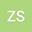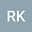On the Exponential Diophantine Equation (aⁿ-2)(bⁿ-2)=x²
••• zafer ŞİAR,
• Refik Keskin
zafer ŞİAR
Bingöl University
Author ProfileRefik Keskin
Sakarya University
Author Profile## Abstract

In this paper, we deal with the equation (aⁿ-2)(bⁿ-2)=x²,2≤a3.1 We solve the equation (3.1) for (a,b)∈{(2,10),(4,100),(10,58),(3,45)}. Moreover, we show that (aⁿ-2)(bⁿ-2)=x² has no solution n,x if 2|n and gcd(a,b)=1. We also give a conjecture which says that the equation (2ⁿ-2)((2P_{k})ⁿ-2)=x² has only the solution (n,x)=(2,Q_{k}), where k>3 is odd and P_{k},Q_{k} are Pell and Pell Lucas numbers, respectively. We also conjecture that if the equation (aⁿ-2)(bⁿ-2)=x² has a solution n,x, then n≤6.